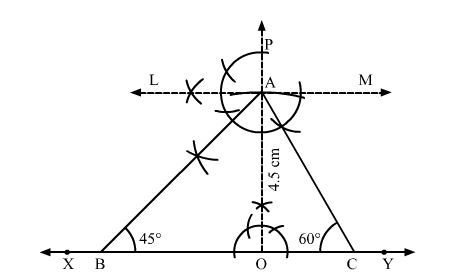# Construct a ∆ABC in which ∠B = 45°, ∠C = 60° and the perpendicular from the vertex A to base BC is 4.5 cm.

Question:

Construct a ∆ABC in which ∠B = 45°, ∠C = 60° and the perpendicular from the vertex A to base BC is 4.5 cm.

Solution:Steps of construction:
1. Draw a line segment XY.
2. Take a point O on XY and draw PO "> XY.
3. Along PO, set off OA = 4.5 cm.

4. Draw a line LM $\| X Y$.

5. Draw $\angle L A B=45^{\circ}$ and $\angle M A C=60^{\circ}$, meeting $X Y$ at $B$ and $C$, respectively.

Thus, ABC is the required triangle.